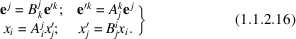International
Tables for
Crystallography
Volume D
Physical properties of crystals
Edited by A. Authier

International Tables for Crystallography (2006). Vol. D, ch. 1.1, p. 6

## Section 1.1.2.4.1. Covariant coordinates

A. Authiera*

aInstitut de Minéralogie et de la Physique des Milieux Condensés, Bâtiment 7, 140 rue de Lourmel, 75015 Paris, France
Correspondence e-mail: aauthier@wanadoo.fr

#### 1.1.2.4.1. Covariant coordinates

| top | pdf |

Using the developments (1.1.2.1)and (1.1.2.5), the scalar products of a vector x and of the basis vectorscan be written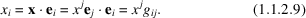The n quantitiesare called covariant components, and we shall see the reason for this a little later. The relations (1.1.2.9)can be considered as a system of equations of which the components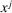are the unknowns. One can solve it since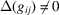(see the end of Section 1.1.2.2). It follows that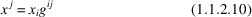with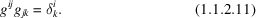The table of the's is the inverse of the table of the's. Let us now take up the development of x with respect to the basis: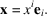Let us replaceby the expression (1.1.2.10):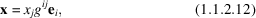and let us introduce the set of n vectors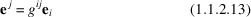which span the space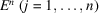. This set of n vectors forms a basis since (1.1.2.12)can be written with the aid of (1.1.2.13)as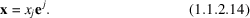The's are the components of x in the basis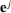. This basis is called the dual basis. By using (1.1.2.11)and (1.1.2.13), one can show in the same way that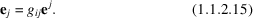It can be shown that the basis vectorstransform in a change of basis like the componentsof the physical space. They are therefore contravariant. In a similar way, the componentsof a vector x with respect to the basistransform in a change of basis like the basis vectors in direct space,; they are therefore covariant: